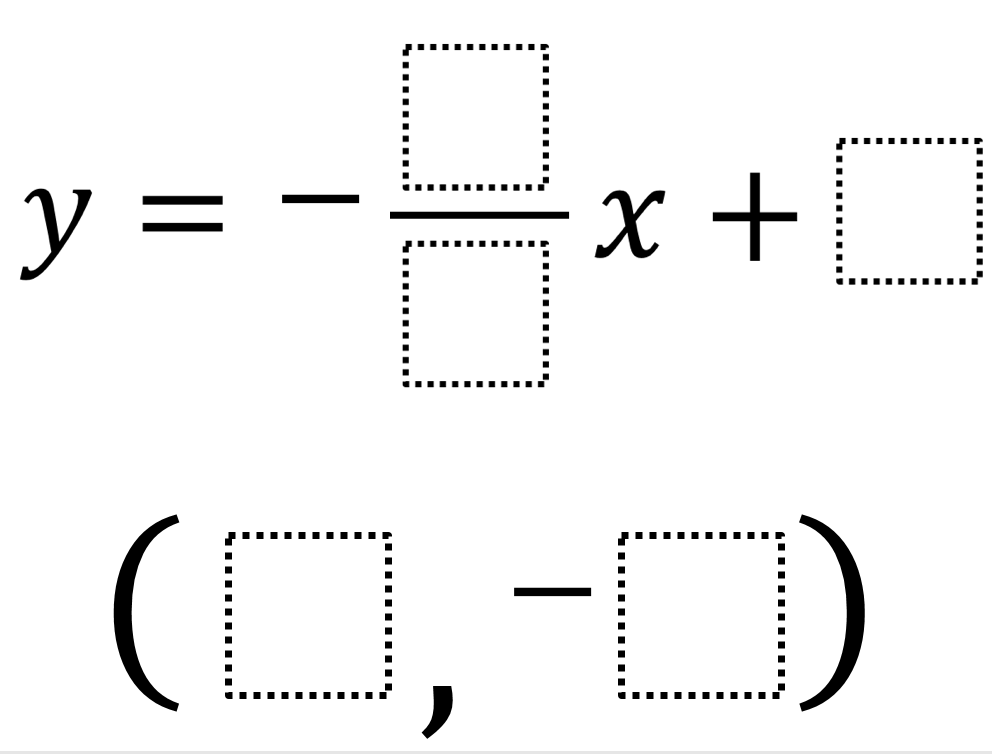# Max Intercept

Directions: Using the whole numbers 1 through 9, no more than one time each, write the equation of a line that passes through the point with the largest possible y-intercept. How many solutions can you find?### Hint

For a given y-intercept, how do you figure out a possible slope and point?

17 possible solutions that have 9 as the y-intercept:
y=-8/1x+9 through (2, -7)
y=-7/1x+9 through (2, -5)
y=-6/1x+9 through (2, -3)
y=-5/1x+9 through (3, -6)
y=-8/2x+9 through (4, -7)
y=-3/1x+9 through (5, -6)
y=-6/2x+9 through (4, -3)
y=-4/2x+9 through (5, -1)
y=-6/3x+9 through (5, -1)
y=-8/4x+9 through (5, -1)
y=-2/1x+9 through (6, -3)
y=-4/2x+9 through (6, -3)
y=-8/4x+9 through (6, -3)
y=-2/1x+9 through (7, -5)
y=-4/2x+9 through (7, -5)
y=-6/3x+9 through (7, -5)
y=-8/4x+9 through (7, -5)

Source: Andy Schwen

## Square Root Expression

Directions: Use the digits 1 to 9, at most one time each, to fill in …

1.2.3.